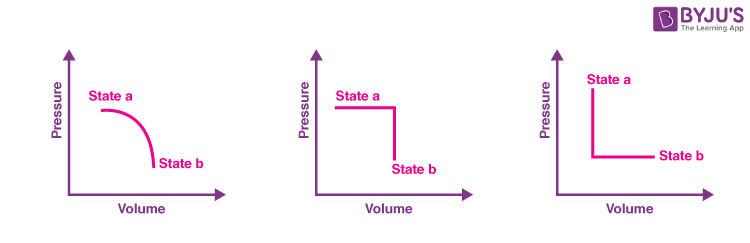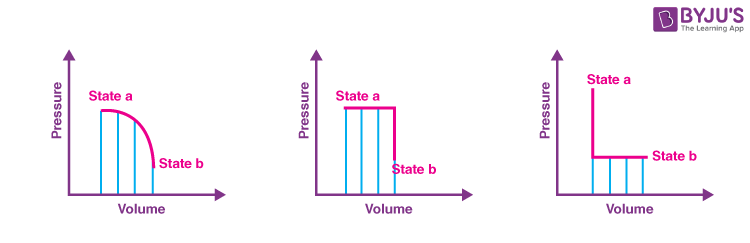# Introduction To Heat, Internal Energy And Work

We already know what heat is. It is a form of energy but it always comes into the picture when energy is being transferred from one system to another. Suppose we are at an initial state ‘a’ and want to go to a final state ‘b’. We can do that by various processes (as shown in the figure) and the heat energy released or absorbed in all the processes is different. So we can see that heat does not depend on the state of the system.So there was a need to define another term that was dependent on the state of the system. This is termed as Internal Energy. In the next section, let us learn more about internal energy.

## What is Internal Energy?

Internal energy definition is given as:

The energy contained within the system associated with random motions of the particles along with the potential energies of the molecules due to their orientation.

The energy due to random motion includes translational, rotational, and vibrational energy. It is represented as U. So now we can say, since internal energy is a state function and in all the processes shown above the change in internal energy from state, ‘a’ to state ‘b’ will be the same.

### Internal Energy Formula

The following is the formula for internal energy:

 ΔU = Q + W

Where,

• ΔU is the internal energy
• Q is the heat added to the system
• W is the work done by the system

### Difference between Internal Energy and Enthalpy

Interval energy vs enthalpy is given below:

 Internal energy Enthalpy It is the total energy in the system It is the relationship between the system and the surrounding The formula of internal energy is: ΔU = Q + W The formula of enthalpy is: H = U + PV

### Relation between Internal Energy and Enthalpy

The following derivation is the explanation for the relation between internal energy and enthalpy for an ideal gas, also a mathematical way to show that the internal energy of an ideal gas is a function of temperature only.

For an ideal gas, the internal energy is given as:

U = U(T)

And enthalpy is given as:

H = U + PV

Using the ideal gas equation, PV = RT

H = U + RT

In the above equation, R is the universal gas constant.

Enthalpy of an ideal gas is given as:

H = H(T)

We know that specific heats at constant pressure and volume are temperature-dependent and can be given as:

dU = Cv (T) dT

dH = Cp (T) dT

Using above equations, specific heat ratio k is given as:

$$\begin{array}{l}k= \frac{C_{p}}{C_{v}}=\frac{U}{H}\end{array}$$

This is the relationship between internal energy and enthalpy for an ideal gas.

### Work Done by a System

Similar to the quantities heat and internal energy, there is another term known as work that is associated with the transfer of energy. Suppose a piston contains gas. If the piston moves outwards, we say work is done by the gas and it is positive. Similarly, if it moves inwards, work is done on the gas and it is negative. Let’s take the following figure:$$\begin{array}{l}Work\,\, done = \int(F.dx) = \int P(A.dx) = \int P.dV \end{array}$$

We can use the above equation to find the work done by gas. Also, the graphical approach suggests that work done is the area under the P–V graph. So for the above-described process, we can see the area is different for different processes (as shown in the figure below), and hence work is also not a state function and depends on the path.## Frequently Asked Questions – FAQs

Q1

### What is internal energy?

The energy contained within the system associated with random motions of the particles along with the potential energies of the molecules due to their orientation is known as internal energy.
Q2

### What is thermodynamics?

Thermodynamics in physics is a branch that deals with heat, work and temperature, and their relation to energy, radiation and physical properties of matter.
Q3

### What is entropy?

Entropy is a thermodynamic quantity whose value depends on the physical state or condition of a system. In other words, it is a thermodynamic function used to measure randomness or disorder.
Q4

### What is heat?

With the increase in the temperature of a body, the vibrations of molecules or atoms increase. These vibrations are then transferred from one part of the body to another. The measure of energy with which the molecules vibrate in a system is termed as heat stored in that object.
Q5

### Define joule.

The SI unit of work is joule (J), which is defined as the work done by a force of 1 Newton in moving an object through a distance of 1 metre in the direction of the force.
Test your knowledge on Internal energy and work